# MAP 7th Grade Math : Operations and Algebraic Thinking

## Example Questions

### Example Question #1 : Operations And Algebraic Thinking

Elizabeth is going to buy concert tickets. The concert tickets costs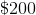plus asales tax. What is the total price that Elizabeth will pay for her concert tickets?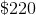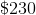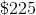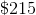Explanation:

We know that Elizabeth is going to pay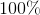of theconcert because that is the total cost. Plus, she is going to payof thebecause that is the sales tax.

First, we need to convert our percentages into decimals in order to multiply.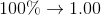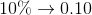Next, we can write a numeric expression and solve: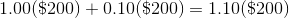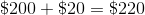### Example Question #2 : Operations And Algebraic Thinking

Billy makes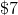a week in allowance plus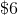for each lawn that he mows. This week Billy wants to make over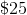. Select the inequality for the number of lawns he needs to mow.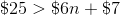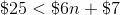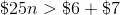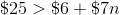Explanation:

We know that Billy needs to make more thanbetween his allowance and the lawns that he mows. This means our inequality should include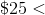Also, since Billy will makeper lawn, that means we need to multiplyby the number of lawns he needs to mow,: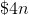So far we have the following: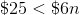Next, we know that he makeseach week, on top of what he makes mowing each law.

This means we need to add theto the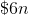When we put all of these pieces together, we will get the following inequality:### Example Question #3 : Operations And Algebraic Thinking

Hannah loves to shop. She can shop inof the mall inof an hour. If she continues at this rate, how much of the mall can she shop per hour?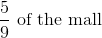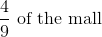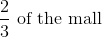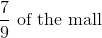Explanation:

The phrase "per hour" gives us a clue that we are going to divide. In this problem, we can replace the word "per" with a division sign; therefore, we will have the portion of the mall,, divided by hours,: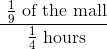Remember that when we divide fractions, we can simply multiply by the reciprocal of the denominator to solve.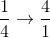Therefore: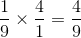Hannah can shop in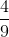of the mall per hour.

### All MAP 7th Grade Math Resources